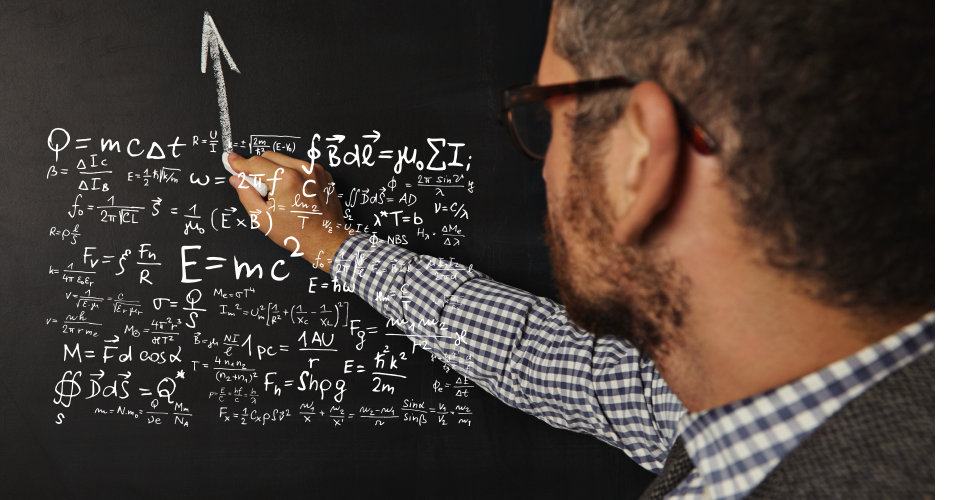• Save

# SSC CGL Maths Questions Bank 2021

Vikram SinghLast week 210 Views
NEWQ :

A container in the shape of a frustum of a cone having diameters of its two circular faces as 35 cm. and 30 cm and vertical height 14 cm. is completely filled with oil. If each cm3 of oil has mass 1.2g, then find the cost of oil in the container if its costs Rs. 40 per kg.

(A) Rs. 597.50

(B) Rs. 540.20

(C) 569.60

(D) Rs. 558.80

Q :

Let ABCD be a quadrilateral. Let the diagonals AC and BD meet at O. Let the perpendicular drawn from A to CD meet CD at E. Further, AO: OC = BO: OD, AB = 30 cm. CD = 40 cm. and the area of the quadrilateral ABCD is 1050 cm2. What is BE equal to?

(A) 30√2

(B) 40√2

(C) 60√2

(D) 20√2

Q :

A kite is flying at a height of 50m. If the length of string is 100m then the inclination of string to the horizontal ground in degree measures is:

(A) 90

(B) 45

(C) 60

(D) 30

Q :

What is the value of $${\sqrt{1-cosA\over{1+cosA}}}$$?

(A) cosecA-sinA

(B) cosecA+cotA

(C) secA-cotA

(D) secA+cotA